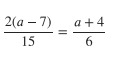PSABM5ZA - Practice---Acc7.5 Lesson 18 What a Point in a Scatter Plot Means (5.G.A.2, 8.SP.A.1, 8.SP.A.3)
Part A)

Here is a table and a scatter plot that compares points per game to free throw attempts for a basketball team during a tournament.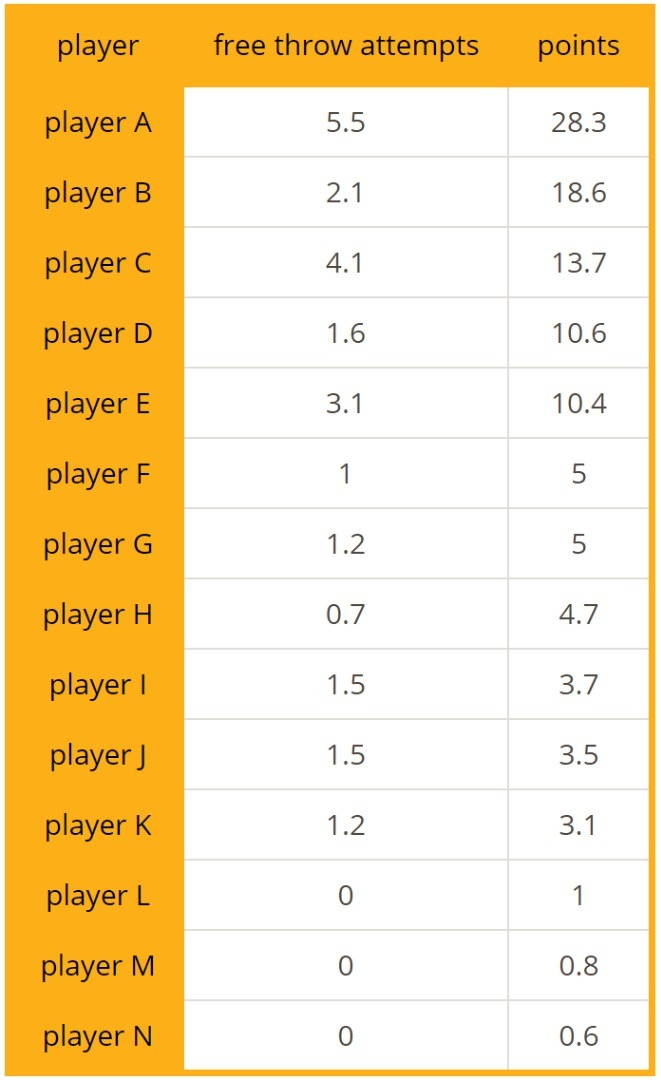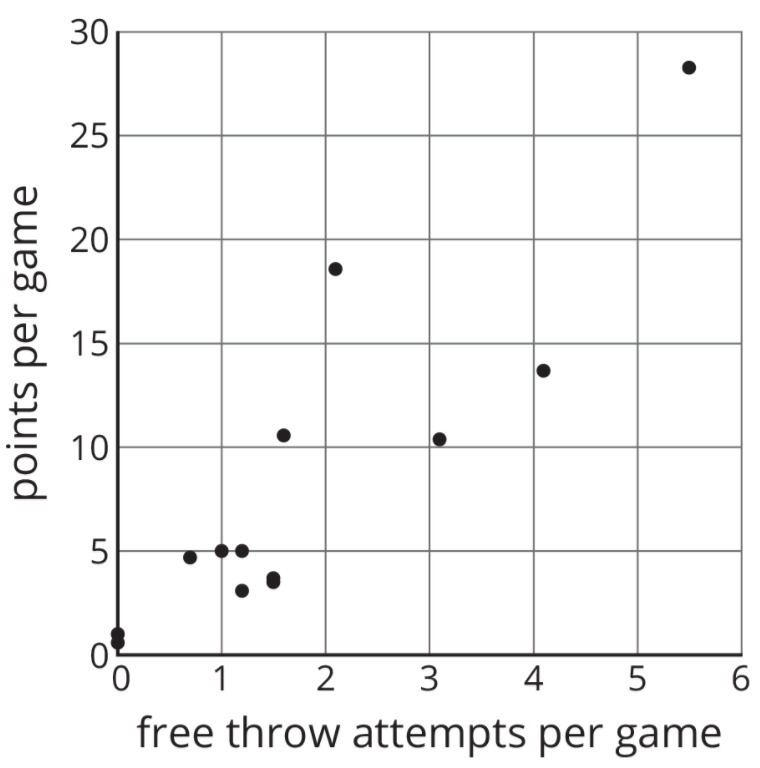Circle the point that represents the data for Player E.

Indicate the point on paper, take a picture, and upload it using the image upload icon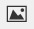.

If you do not have the ability to upload an image of your work type "Point indicated on paper."

Part B)

What does the point (2.1,18.6) represent?

Part C)

In that same tournament, Player O on another team scored 14.3 points per game with 4.8 free throw attempts per game. Plot a point on the graph that shows this information.

Draw your graph with the new point on paper, take a picture, and upload it using the image upload icon.

If you do not have the ability to upload an image of your work type "Graph is on paper."

Andre said, “I found two figures that are congruent, so they can’t be similar.” Diego said, “No, they are similar! The scale factor is 1.” Do you agree with either of them? Use the definition of similarity to explain your answer.

Part A)

For each equation, explain what you could do first to each side of the equation so that there would be no fractions. You do not have to solve the equations (unless you want more practice).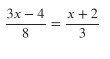Part B)

For each equation, explain what you could do first to each side of the equation so that there would be no fractions. You do not have to solve the equations (unless you want more practice).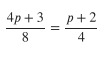Part C)

For each equation, explain what you could do first to each side of the equation so that there would be no fractions. You do not have to solve the equations (unless you want more practice).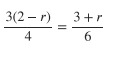Part D)

For each equation, explain what you could do first to each side of the equation so that there would be no fractions. You do not have to solve the equations (unless you want more practice).